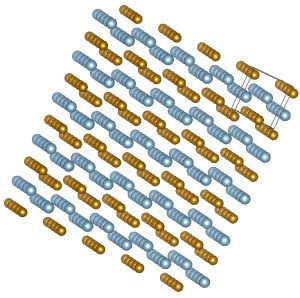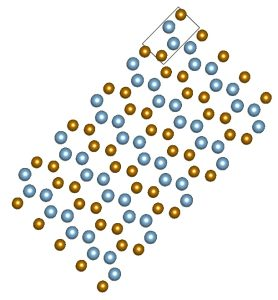### Computational Materials Physics

For this chapter, there is one continuous exercise that explains all steps of the geometry optimization process. Instructions for this exercise are given after each of the following videos. Do these exercise, and keep track of your experiences and results in a document of your choice. At the end of the chapter you can convert this to pdf, and upload it.

The starting point of this exercise is a hypothetical crystal for which you have the cif file here. It is based on an ideal hexagonal close-packed crystal, which is the crystal structure of orderly stacked identical hard spheres (oranges, canon balls,…– watch this optional video about close-packing if you want to know more). The unit cell of a hexagonal closed packed crystal has lattice parameters a=b and c, and cell angles α=β=90º and γ=120º. In an ideal hexagonal closed packed unit cell, the ratio c/a=√(8/3)≈1.633.

In an ideal close-packed crystal, all spheres are identical. In our hypothetical crystal, all spheres are identical in size, yet ‘coloured’ in two different ways: half of them are Fe-atoms, the other half are Al-atoms. The choice which sphere is Fe and which is Al is not made randomly, yet in a particular ordered way. The presence of these colours breaks the hexagonal symmetry, but due to the chosen ordered way of colouring, not all symmetry has gone: our hypothetical crystal has orthorhombic symmetry: all three cell edges are unequal in length, while all cell angles are 90°. Its lattice parameter a corresponds to the a=b of the hexagonal cell. Its lattice parameter b corresponds to the c of the hexagonal cell (check in the cif file that b/a is indeed 1.633). Its lattice parameter c is √3 times the lattice parameter a of the hexagonal cell. This orthorhombic cell is has only one degree of freedom: as soon as the lattice parameter a is specified, everything is known. In the cif file given above, the lattice parameter a is chosen such that the volume per atom in this cell is the average of the volume per atom in fcc-Al and bcc-Fe.

Visualize this cif file with Vesta or another program, play with it, and familizarize yourself with this crystal structure. Some snapshots are here:This is just a starting point. Even if someone in a lab would succeed to bring all these atoms exactly at these positions, chances are rare that they will want to stay there. All kinds of optimizations will happen, driven by the desire to lower the total energy of the crystal. In this chapter, we’ll examine these optimizations one by one. You may start by converting this cif file to a format for your favourite DFT code. We will use the PBE-functional.

For Quantum Espresso, use this PAW pseudopotential for Fe and this PAW pseudopotential for Al. For medium precision calculations, use ecutwfc=70 Ry, ecutrho=700 Ry, k-mesh=6x4x4. For high precision calculations (which take more computer time), use ecutwfc=90 Ry, ecutrho=900 Ry, k-mesh=14x8x8. It will always be indicated when it is safe to use medium precision.

The guidelines on the following pages assume calculations without magnetism (nspin=1).

Do you run into the “***Warning: Space group operation check failed for Hall symbol” error when converting this cif file using cif2cell? Here is the solution.

Scroll to Top